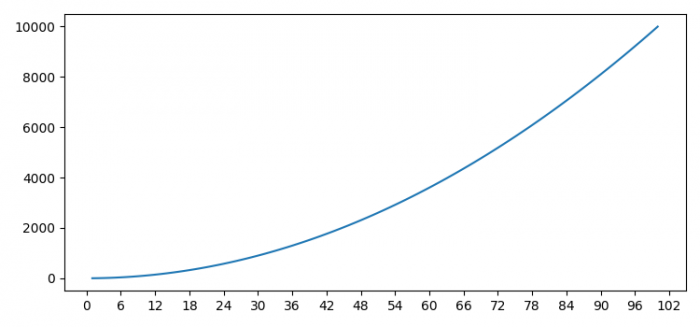# How to set the value of the axis multiplier in matplotlib?

To set the value of the axis multiplier in matplotlib, we can take the following steps −

## Steps

• Set the figure size and adjust the padding between and around the subplots.

• Create x data points using numpy.

• Plot x and x2 using plot() method.

• Get the current axis of the figure.

• Initialize a variable multiplier, i.e., a value of the axis multiplier.

• Set a tick on each integer multiple of a base within the view interval.

• Set the locator of the major ticker.

• To display the figure, use show() method.

## Example

# Import matplotlib and numpy
from matplotlib import pyplot as plt
import numpy as np

# Set the figure size
plt.rcParams["figure.figsize"] = [7.50, 3.50]
plt.rcParams["figure.autolayout"] = True

# Create x data points
x = np.linspace(1, 100, 100)

# Plot x and x2
plt.plot(x, x**2)

# Get the current axis
ax = plt.gca()

# Axis multiplier
multiplier = 6

# Set a tick on each integer multiple
locator = plt.MultipleLocator(multiplier)

# Set the locator of the major ticker
ax.xaxis.set_major_locator(locator)

plt.show()

## Output

It will produce the following output −